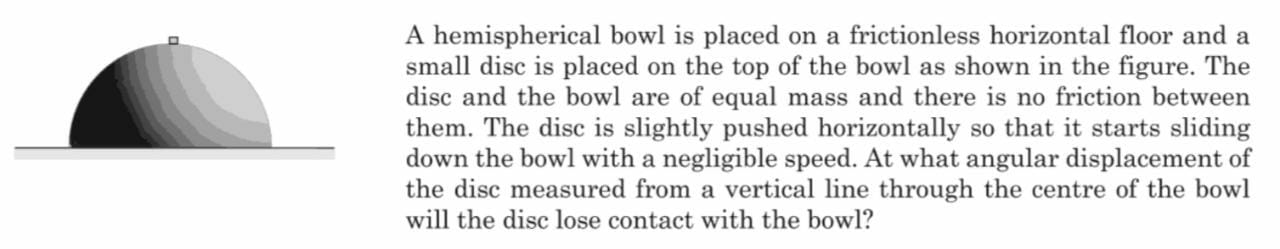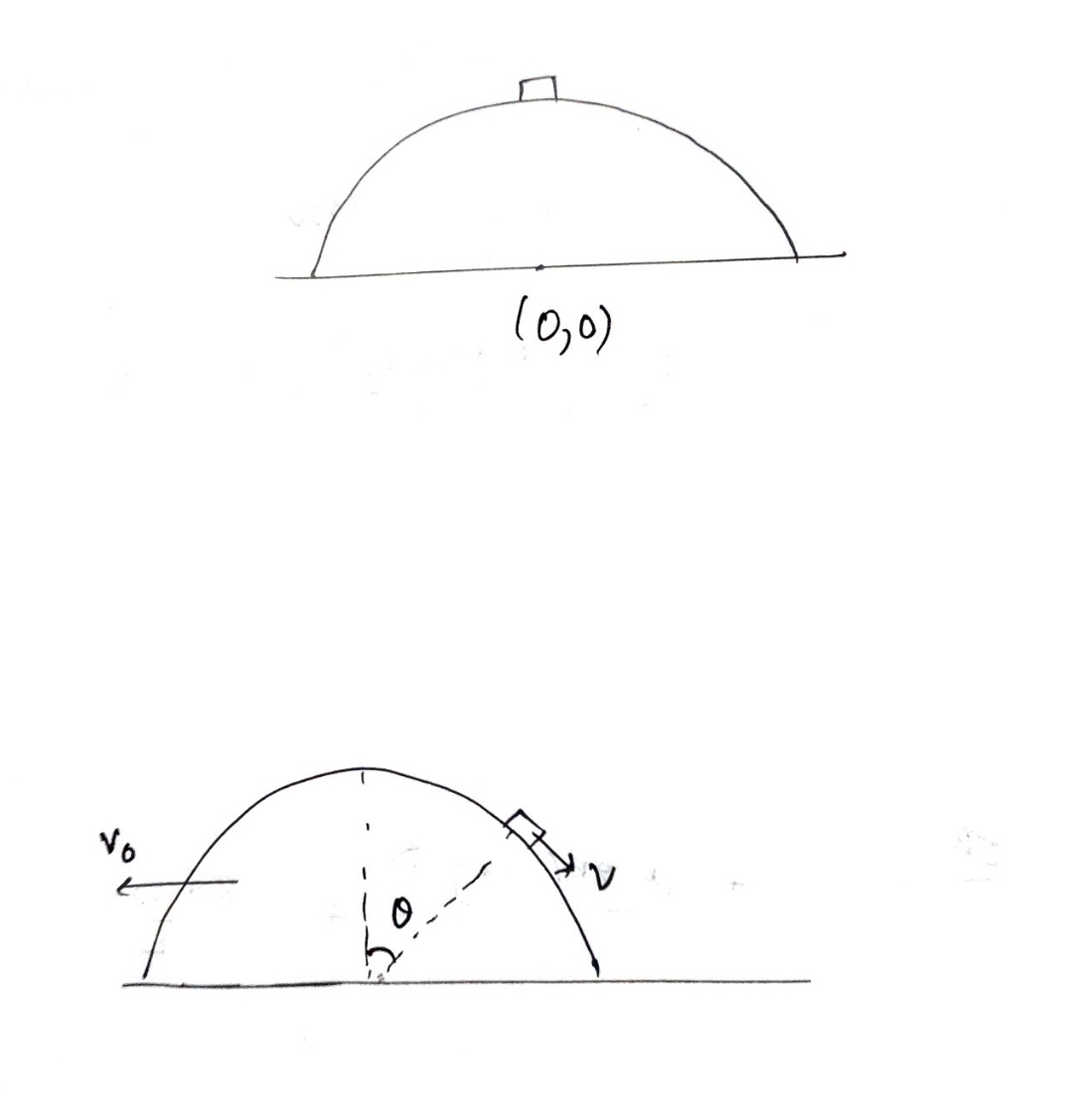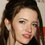# An interesting COM problem

I was solving a problemHere is the solutionThe velocity of that small disc $v$ is with respect to hemisphere frame .
And the velocity of that $v_{0}$ of that hemisphere is in ground frame.

So, $F_{x} =0$
So let's conserve momentum in $x$ direction $0=-mv_{0}+m(v \cos \theta -v_{0})$ After this conserving energy as always $mgR=\frac{1}{2}mv_{0}^{2}+\frac{1}{2}m(v_{0}^{2}+v^{2}+2vv_{0} \cos (π- \theta))+ mg(R-R \cos \theta)$
And the last equation normal to the inclined plane when it losses contact normal reaction will be zero $N=0$
Which gives the equation $\frac{mv^{2}}{R}=mg \cos \theta$
After solving these 3 equations patiently gives $\cos \theta= \sqrt{3}-1, 2,-1-\sqrt{3}$
The only reasonable value of $\cos \theta$ seems to be $\large \boxed{cos \theta=\sqrt{3}-1}$Note by Talulah Riley
9 months ago

This discussion board is a place to discuss our Daily Challenges and the math and science related to those challenges. Explanations are more than just a solution — they should explain the steps and thinking strategies that you used to obtain the solution. Comments should further the discussion of math and science.

When posting on Brilliant:

• Use the emojis to react to an explanation, whether you're congratulating a job well done , or just really confused .
• Ask specific questions about the challenge or the steps in somebody's explanation. Well-posed questions can add a lot to the discussion, but posting "I don't understand!" doesn't help anyone.
• Try to contribute something new to the discussion, whether it is an extension, generalization or other idea related to the challenge.
• Stay on topic — we're all here to learn more about math and science, not to hear about your favorite get-rich-quick scheme or current world events.

MarkdownAppears as
*italics* or _italics_ italics
**bold** or __bold__ bold
- bulleted- list
• bulleted
• list
1. numbered2. list
1. numbered
2. list
Note: you must add a full line of space before and after lists for them to show up correctly
paragraph 1paragraph 2

paragraph 1

paragraph 2

[example link](https://brilliant.org)example link
> This is a quote
This is a quote
    # I indented these lines
# 4 spaces, and now they show
# up as a code block.

print "hello world"
# I indented these lines
# 4 spaces, and now they show
# up as a code block.

print "hello world"
MathAppears as
Remember to wrap math in $$ ... $$ or $ ... $ to ensure proper formatting.
2 \times 3 $2 \times 3$
2^{34} $2^{34}$
a_{i-1} $a_{i-1}$
\frac{2}{3} $\frac{2}{3}$
\sqrt{2} $\sqrt{2}$
\sum_{i=1}^3 $\sum_{i=1}^3$
\sin \theta $\sin \theta$
\boxed{123} $\boxed{123}$

## Comments

Sort by:

Top Newest

@Karan Chatrath @Steven Chase @Krishna Karthik in case you guys are interested.

- 9 months ago

Log in to reply

Hmmm nice one

- 9 months ago

Log in to reply

@Krishna Karthik,mention[6497226:Krishna Karthik]

- 9 months ago

Log in to reply

@SRIJAN Singh Yes; what do you need?

- 9 months ago

Log in to reply

×

Problem Loading...

Note Loading...

Set Loading...